# Bernoulli numbers

(diff) ← Older revision | Latest revision (diff) | Newer revision → (diff)

The sequence of rational numbersdiscovered by Jacob Bernoulli  in connection with the calculation of the sum of equal powers of natural numbers: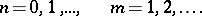The values of the first Bernoulli numbers are:All odd-indexed Bernoulli numbers except for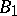are zero, and the signs of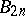alternate. Bernoulli numbers are the values of the Bernoulli polynomials at: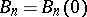; they also often serve as the coefficients of the expansions of certain elementary functions into power series. Thus, for example,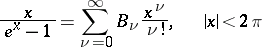(the so-called generating function of the Bernoulli numbers);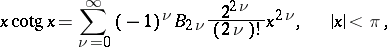L. Euler in 1740 pointed out the connection between Bernoulli numbers and the values of the Riemann zeta-function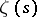for even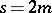:Bernoulli numbers are used to express many improper integrals, such as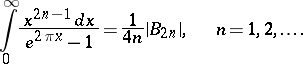Certain relationships involving Bernoulli numbers are: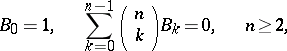(the recurrence formula);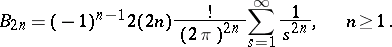The estimates: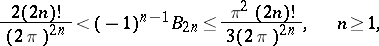hold. Extensive tables of Bernoulli numbers are available; for instance,  contains accurate values offorand approximate values for.

Bernoulli numbers have found many applications in mathematical analysis, number theory and approximate calculations.

How to Cite This Entry:
Bernoulli numbers. Encyclopedia of Mathematics. URL: http://encyclopediaofmath.org/index.php?title=Bernoulli_numbers&oldid=15872
This article was adapted from an original article by Yu.N. Subbotin (originator), which appeared in Encyclopedia of Mathematics - ISBN 1402006098. See original article